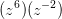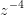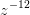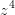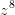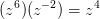# Pre-Algebra : Product Rule of Exponents

## Example Questions

### Example Question #11 : Product Rule Of Exponents

Simplify: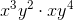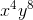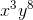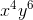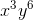Explanation:

Use the product rule of exponents, which states that when multiplying expression(s) with the same base, you add the exponents together: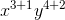Therefore the correct answer is.

### Example Question #12 : Product Rule Of Exponents

Evaluate: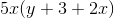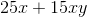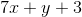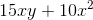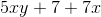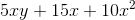Explanation:

Distribute the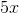to each of the terms and add them.### Example Question #13 : Product Rule Of Exponents

Simplify: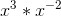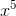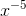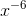Explanation:

The general rule for multiplying terms with exponents is that we add the exponents together. In this case the exponents areand, and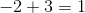.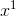is just: anything to the first power is itself.

### Example Question #11 : Product Rule Of Exponents

Simplify: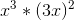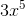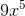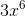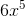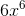Explanation:

To simplifyit helps to understand what it means.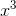just means x times itself 3 times,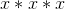.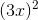means multiplytimes itself, or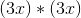. We can simplify that by multiplying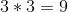and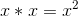. Multiplying both terms together gives us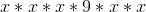. This is a 9 and 5 x's being multiplied together, or.

### Example Question #11 : Product Rule Of Exponents

Simplify: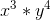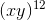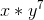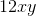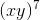Explanation:

The product rule tells us that if we're multiplying 2 terms with exponents, we add the exponents. However, that is only applicable for 2 terms with the same base. In this case, our bases are different variables, so we can't use the product rule. This expression is as simplified as possible.

### Example Question #13 : Product Rule Of Exponents

Use the product rule of exponents to simplify: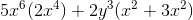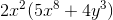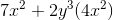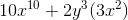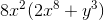Explanation:

The product rule states that when two like bases with the same power are being multiplied, we add the powers together and preserve the base. In mathematical language,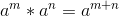. If constants are present in front of either base we multiply them as usual.Combine using the power rule: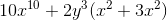Combine the parenthesis: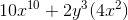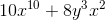Factor fully:### Example Question #15 : Product Rule Of Exponents

Simplify the following expression: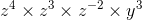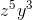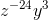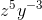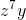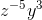Explanation:

The product rule of exponents states that we can multiply two exponents with the same base by adding the exponents. The exponents of different bases cannot be summed, therefore ourterm will not change.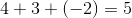Therefore, the simplest form of the expression is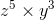which can be written as### Example Question #111 : Polynomials

Simplify: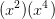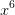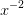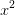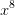Explanation:

When you multiply exponents with the same base, you add the exponents: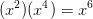### Example Question #11 : Product Rule Of Exponents

Simplify: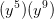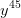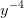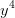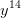Explanation:

When you multiply exponents with the same base, you add the exponents: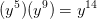### Example Question #20 : Product Rule Of Exponents

Simplify: